# Handwriting Programs in J

Posted on

I think by hand. It’s easier for me to write my first drafts on a tablet and type them up afterwards. I can’t do this with code, though. Here’s me scrawling out a python function as fast as possible: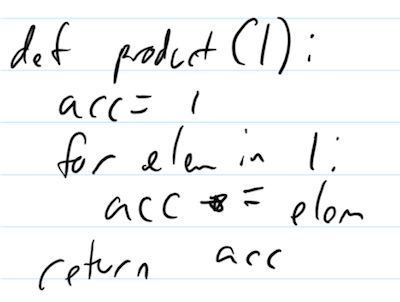That took three times longer to write than type. Something about code being optimized for legibility and IDE usage and lame stuff like that. I still like the idea of writing code, though, so I looked for a language that wasn’t just easy to write, but benefited from being hand-written.

And that brought me down the rabbit hole of J.

## J Orientation

J is an array programming language, designed to process data as fast and as concisely as possible. It’s a descendant of APL and shares the same design philosophy. Since it has a different family tree than most mainstream programming languages, it uses different terminology than you’re probably used to. Instead of functions, we have verbs. Unary verbs are called monads, while binary verbs are called dyads.1 There are no verbs with more than two arguments. All J programs are evaluated right to left.

``````   6 - 1 = 5 NB. This is a comment
6
(6 - 1) = 5
1
``````

In the first case, J evaluates `1 = 5`, which is false, or `0`. Then `6 - 0` is 6. We can also define our own verbs:

``````   mean1 =: verb define
(+/ y) % (# y)
)
mean1 i. 100 NB. 0 2 3 ... 99
49.5
``````

When defining verbs, we always use `y` for the monadic argument and `x` for the (optional) dyadic argument. `# y` is the length of the array `y`.2 `+/ y` is “reduce `y` with `+`”, aka the sum of the elements of y. `x % y` divides x by y. This calculates the mean.

`mean1` is an explicit verb. J programmers often prefer to write tacit verbs, which don’t reference their operands. This is how we’d write mean as a tacit verb:

``````   mean2 =: +/ % #
mean2 i.100
49.5
``````

`mean2` has a fork, where three independent verbs are in sequence. `(f g h) y` expands to `(f y) g (h y)`, turning `g` into a dyad.3 In many cases, the J interpreter can do optimizations on the tacit verbs that it can’t on explicit verbs. Our tacit mean is faster and uses less space for arrays of a thousand elements, although our explicit mean pulls ahead for arrays of ten thousand.

J is full of ways of compacting your scripts. `x f@:g y` expands to `f (x g y)`. `(f g) y` expands to `y f (g y)`. `f \ y` applies `f` to all prefixes of y, such that `f /\ y` becomes accumulate f over y:

``````   +/\ i.10
0 1 3 6 10 15 21 28 36 45
``````

## J Disorientation

You can probably see the problem here: J is fast and concise but also unreadable. It’s not just that the commands look like line noise, it’s that J programs are actually compressions of hideously-complicated call trees. This is what drew me to the language: its complexity is poorly served by monospaced characters. I wondered if freehand would be better.

The key insight to tacit J is that since all verbs are either monads or dyads, every primitive operation can be expressed as a binary tree. And while explicit verbs can have intermediate state, primitives cannot. This means that all tacit verbs expand to binary trees.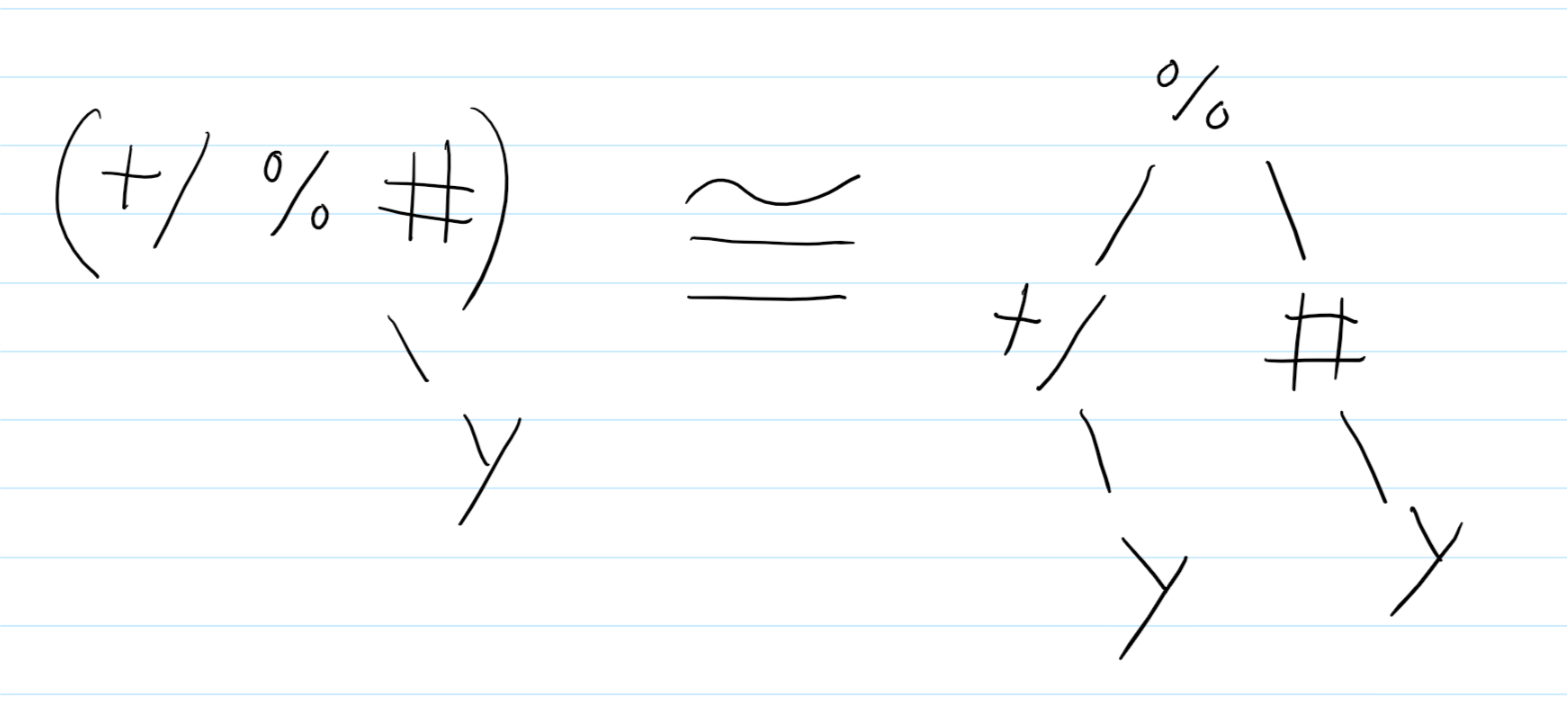This goes both ways: given a binary tree of verbs and nouns, we can (almost always) condense it to a single tacit verb. We know we’re done when our binary tree has a tacit verb as the root node, `y` as its right child, and `x` as its optional left child.

Let’s do an example. I want to write a compound interest simulator, where the annual rate per year can vary with the market. As one step in the process, I need N weighted random numbers to represent return rates per year. I also want to run M simulations at once. That means I want a verb that takes a shape and an array of rates and return a suitable M x N matrix:

``````   3 4 random_matrix 'aaabbc'
aacb
acbb
baba
``````

• `\$` is the “shape” verb. If `x` is an array of dimensions and `y` is a number, then `x` is a matrix of that shape filled with the number.4 If `y` is an array, it will cycle the array to fit.
``````   x =: (2 3) \$ 3 9
x
3 9 3
9 3 9
``````
• `?` is the “random” verb. `? y` is an integer in `[0, y)`. If y is a matrix then it applies the verb to each cell.
``````   ? x
1 7 0
5 1 4
``````
• `#` is the “length” verb. We’ve seen this before.

• `{` is the “take” verb. `x { y` is the x’th element of y.

``````   x { 'abcdefghijkl'
djd
jdj
``````

With all of these pieces, we can express the problem as “find the length of y, initialize a matrix of shape x filled with `len`, randomize every cell to an integer between 0 and `len`, then use the random numbers as indices against y.” The binary tree for our random matrix verb looks like this: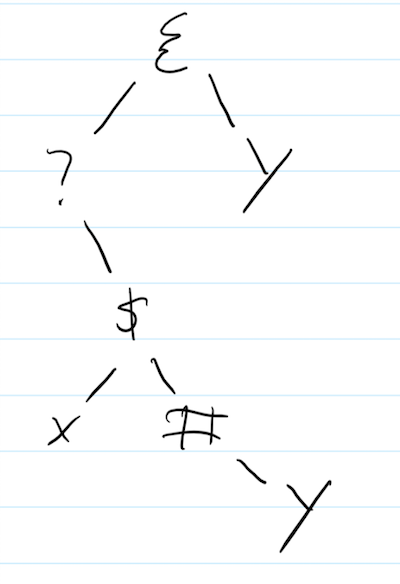``````   explicit =: dyad define
(? x \$ (# y)) { y
)
(3 4) explicit 'aaab'
aaba
aaab
abba
``````

This gives us an explicit verb. However, we don’t yet have a tacit verb: our binary tree is four levels deep. The next step is to use adverbs and conjunctions to transform the tree into a tacit verb.

First, `x \$ (# y)` can be turned into a dyadic hook. While `(f g) y` is `y f (g y)`, as a dyad `x (f g) y` is `x f (g y)`.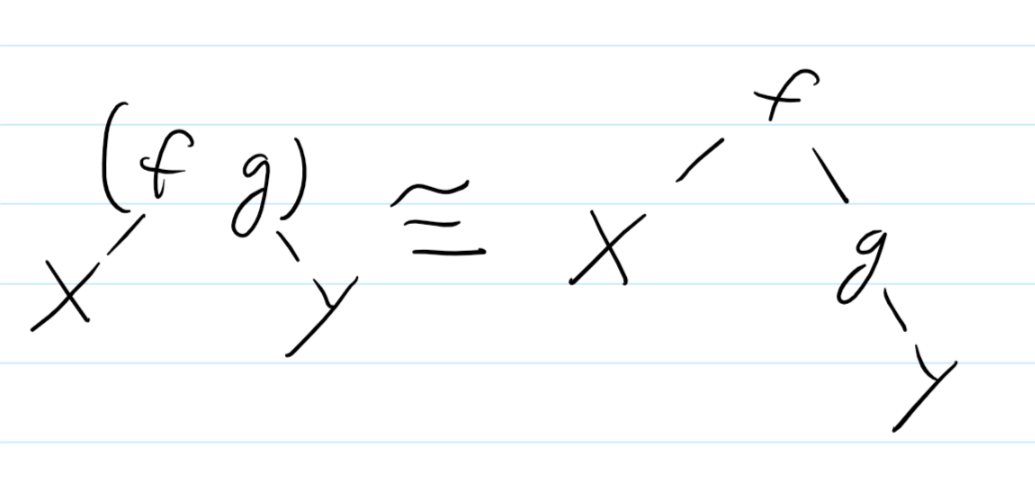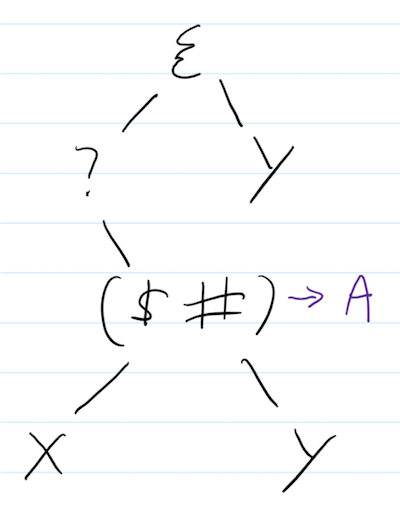Next, we can use the `@:` conjugation. As mentioned before, `x f@:g y` is `f (x g y)`.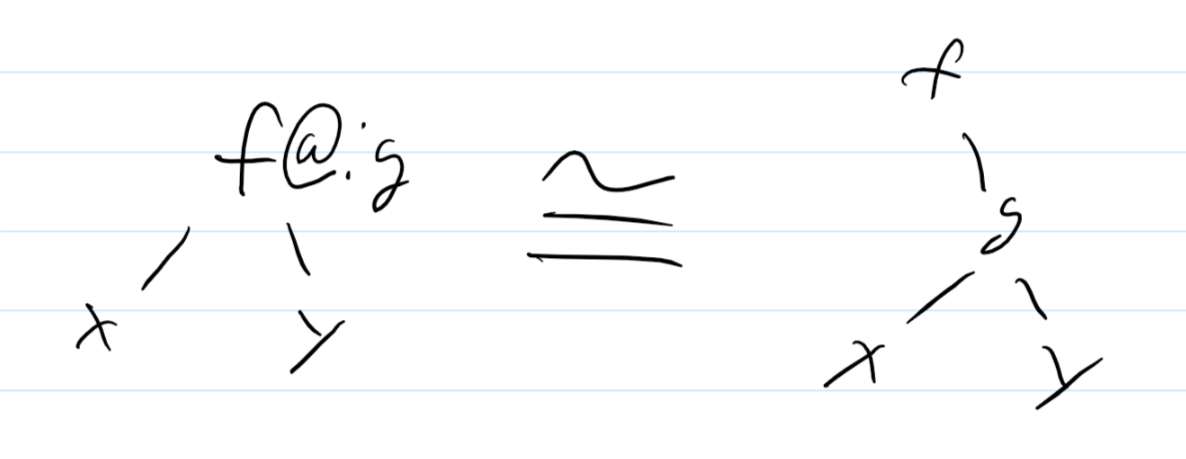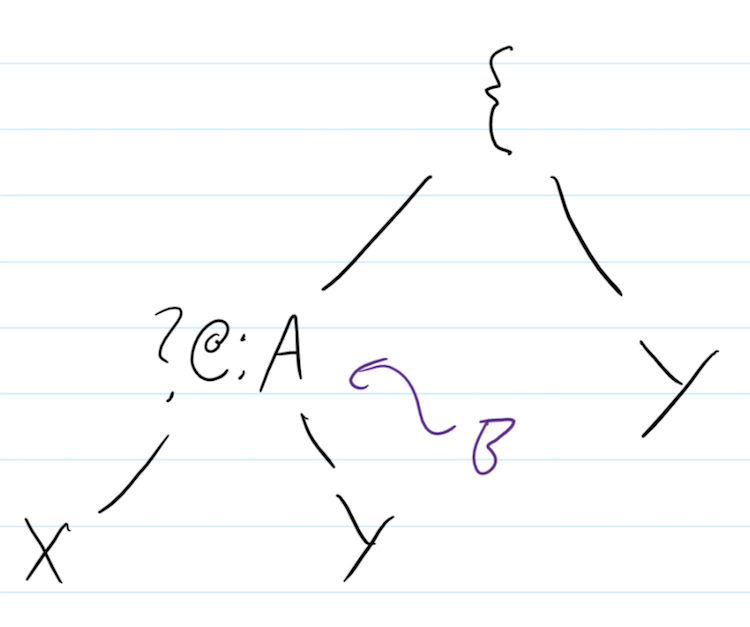Getting closer! This almost looks like a dyadic fork: `x (f g h) y` is `(x f y) g (x h y)`.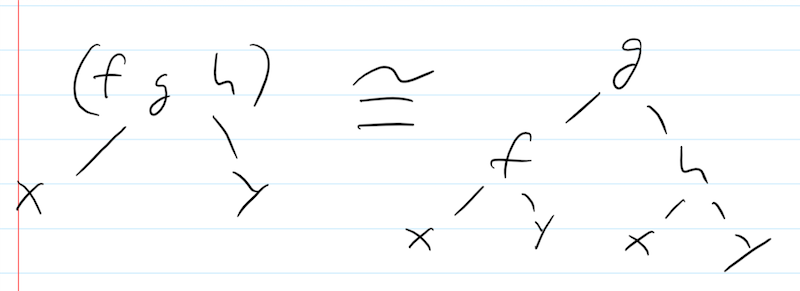However, we only have that on the left side. We could get the right side to match if there was some noop `f` such that `x f y = y`. It wouldn’t affect the computation, but we’d get the right side in the form for a fork. Fortunately, we do have a noop: `]`, or the “right side” verb. `x ] y = y`. Let’s add that.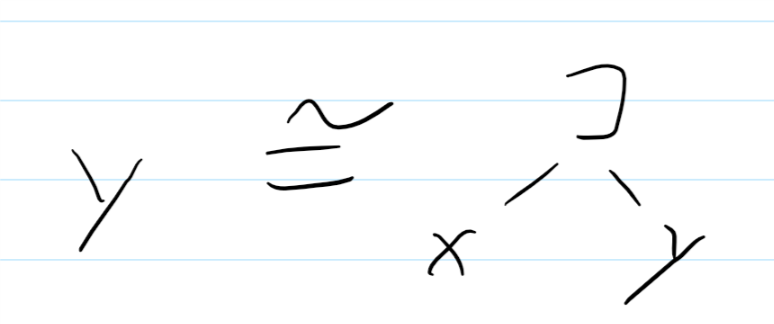And now we can fork!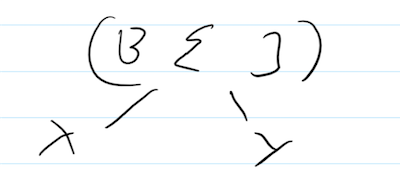Now we have a single verb root with x and y as child nodes. This is a valid tacit verb.

``````   tacit =: (?@:\$ #) { ]
(3 4) tacit 'aaab'
abab
aaaa
baab
``````

We can compare relative performance of the two by using the special `ts` phrase. J code is so concise it’s often easier to copy and paste utility phrases than import entire libraries for them. `ts` will take a sentence and an iteration count and compute the average of the runtime and bytes taken up for space. We’ll average 100 iterations of randomizing a 1000 x 1000 matrix with one of 500 random values:

``````   ts=: 6!:2 , 7!:2@]       NB. Time and space
shape =: 1000 1000
vals =: i. 500
100 ts 'shape explicit vals'
0.0192691 2.51706e7
100 ts 'shape tacit vals'
0.0171166 1.67793e7
``````

Our tacit verb is about 10% faster and uses only two-thirds the total space. Surprisingly, though, we can make both much faster by increasing the size of the value array!

``````   vals =: i. 512
100 ts 'shape explicit vals'
0.00761634 2.51706e7
100 ts 'shape tacit vals'
0.00512317 1.67793e7
``````

Our new explicit is twice as fast as before, because J has special code for picking random numbers in `[0, 2^n)`.5 However, our new tacit is over three times as fast as before! Special codes often synergize in ways that make tacit verbs extremely efficient.

## J Reorientation

We saw being able to sketch our call trees helped us write a tacit verb. But can we also use drawings to read them? I think so. `(?@:\$#){]` Is monospaced, using symbols that don’t carry a lot of semantic meaning. We can use annotations, markups, and different colors to make it more legible. Step one, let’s remove the parenthesis and annotate which verbs form a hook and which form a fork.`@:` is a conjugation; let’s call that out by making it green. We added `]` as a noop, let’s mark that in red:Finally, let’s lower the hook verbs to make the order of evaluation clearer: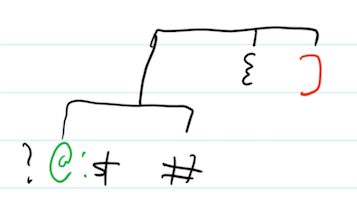We can also use another color as comments, use highlights, etc. I’m still experimenting with what annotations add the most useful information when reading a verb. Here’s an older experiment I tried before settling on marking noops: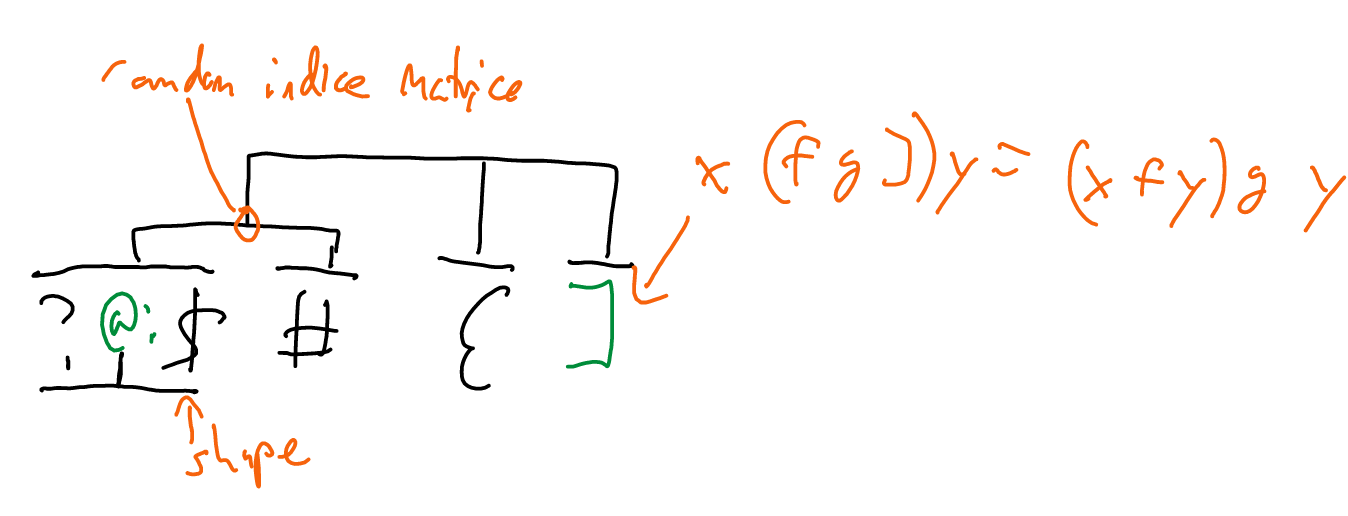## Thoughts

J feels like the perfect language for crunching huge amounts of data. Unfortunately I never have to crunch huge amounts of data and even if I did the syntax would probably scare my coworkers away. Not everybody is as enthusiastic about handwriting everything as I am. So J was a great language to learn from a self-improvement perspective but I don’t see myself doing very much else with it. The financial simulator is coming along pretty well, though!

The real value of J only hit me when I wrote this essay. Sitting on the bus drawing binary tree transformations, marking glyphs in a rainbow of colors and annotating them with lines and curves, I suddenly felt like I was scribing a spell. I got to imagine I was a crazy old wizard. That more than anything else is why I love J. Because if we can’t have fun with programming, why bother?

1. APL was the first language to use “monad” as a term. The popular FP meaning only appeared thirty years later. [return]
2. Like most verbs, `#` works on arbitrary-dimension arrays. If `y` is a matrix, `#` is the row count. [return]
3. `mean2 y` is ‘actually’ `(+/ % #) y`. `f g h y` is just `f (g (h y))`. [return]
4. It’s actually broader than that: it works for any tensor, too. [return]
5. You’re welcome to read the source code if you want to know why. You brave, foolish soul. [return]